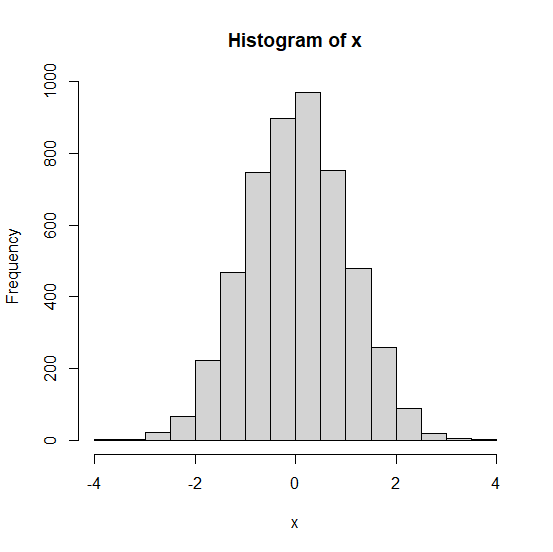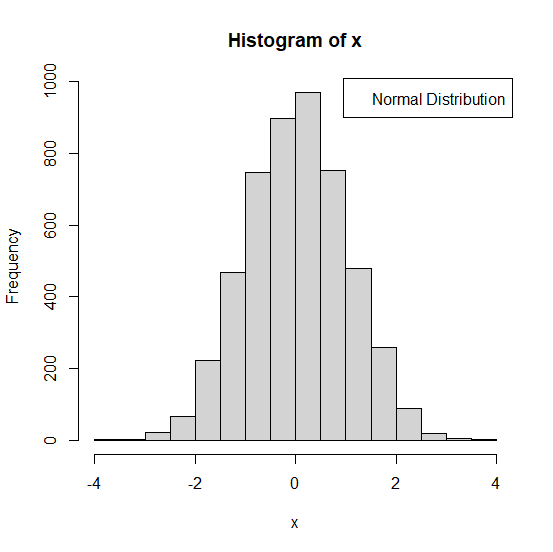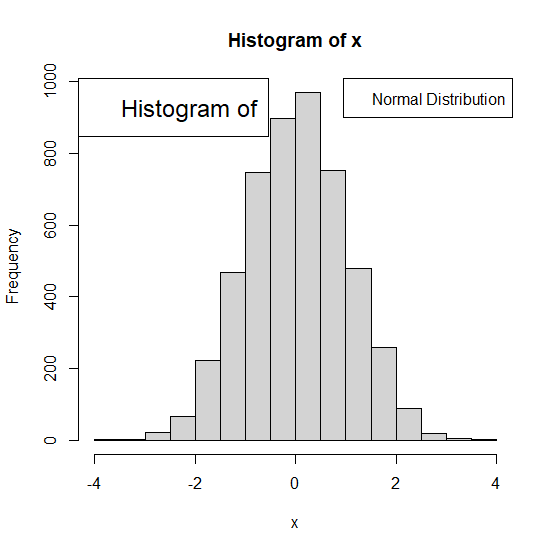# How to change the font size of legend in base R plot?

In base R, we can use legend function to add a legend to the plot. For example, if we want to create a histogram with legend on top-right position then we can use legend("topright",legend="Normal Distribution") and if we want to change the font size then we need to as cex argument as shown below:

legend("topright",legend="Normal Distribution",cex=2)

## Example

Live Demo

Consider the below histogram −

x<−rnorm(5000)
hist(x)

## OutputAdding legend of different sizes −

legend("topright",legend="Normal Distribution",cex=1)


## Outputlegend("topleft",legend="Histogram of",cex=1.5)

## Output